# How to Convert Hours and Minutes to Decimal in Excel (2 Cases)

Get FREE Advanced Excel Exercises with Solutions!

In this tutorial, we will learn how to convert Hours and Minutes to decimal in Excel. While working with time-related data we don’t need to keep the input in time format always. Sometimes we need to convert hours and minutes to decimal values. To illustrate the concept of this article we will show you multiple ways to convert hours and minutes to decimal in Excel.

We will discuss this article for 2 cases. One case is converting hours to decimal and another is converting minutes to decimal. For each case, we will show 3 different ways to convert the time value into decimal.

## Case 1: Converting Hours to Decimal in Excel

In the first case, we will convert the hour’s value to decimal. As previously said, we will demonstrate three distinct methods for converting hours to decimal in this situation.

### 1.1 Using Simple Multiplication to Convert Hours to Decimal in Excel

In the first method, we will convert the hours to decimal values simply by multiplying the original value with 24. To demonstrate this method, we will use the following dataset. The dataset contains the values of six subjects as well as the duration of their class time. We can see the duration of the class in “h:mm: ss” format.So, let’s see the steps to convert hours to decimal values by using simple multiplication.

STEPS:

• Firstly, select cell D5. Insert the following in that cell:
`=C5*24`• Now press Enter. This action returns the time value “1:20:00” into decimal value “1.33”.• Secondly, select cell D5. Move the mouse cursor to the bottom right corner of the selected cell so that it turns into a plus (+) sign like the following image.• Then, click on the plus (+) sign and drag the Fill Handle down to cell D10 to copy the formula of cell D5 in other cells. We can also double-click on the plus (+) sign to get the same result.• Next, release the mouse click.
• So, we can see the converted values of hours into decimal values in cells (D5:D10).• Thirdly, we will convert the decimal value into an integer value with the INT function. To do this select cell E5 and insert the following formula:
`=INT(D5)`• Next, press Enter. This action converts the decimal value “1.33” minutes into an integer value “1”.• Again, select cell E5. Make the plus (+) sign visible by moving the mouse cursor at the bottom right corner of that cell.• Then, click on the plus (+) sign and drag the Fill Handle down to cell E10 or you can do this by double-clicking on the plus (+).• Lastly, we can see the converted integer values cells (E5:E10).Read More: How to Convert Hours to Minutes in Excel

### 1.2 Applying Excel CONVERT Function to Convert Hours to Decimal

In Microsoft Excel, the CONVERT function converts the system of measurement of a number. In this method, we will use the CONVERT function to convert hours to decimal in excel. We will use the same dataset that we have used in the previous example.Now, let’s see the steps regarding this method.

STEPS:

• First, select cell D5. Insert the following formula in that cell:
`=CONVERT(C5,"day","hr")`• Press Enter. This action returns the value of hours of cell C5 in decimal which is “1.33”.• Next, move the mouse pointer to the bottom right corner of cell D5, where it will convert into a plus (+) sign as shown in the image below.• After that, click the plus (+) sign and drag the Fill Handle down to cell D10 to copy the formula from cell D5. You can also get the same result by double-clicking on the plus (+) sign.• Release the mouse button now.
• Finally, the operation above duplicates cell D5’s formula to the other cells. As a result, all the time values in the duration column convert into decimal values.• Then, we’ll turn the decimal value into an integer value. To accomplish this, go to cell E5 and type in the formula:
`=INT(D5)`• Press Enter. This command changes the decimal value of “1.33” minutes to an integer value of 1.• Now, move the mouse cursor to the bottom right corner of cell E5 to make the plus (+) sign visible.• After that, either double-click on the plus (+) sign or click on the plus(+) sign and drag the Fill Handle tool down to cell E10.• Finally, we get the integer values of hours in cells (E5:E10).### 1.3 Combining Excel HOUR, MINUTE, and SECOND Functions to Convert into Decimal

The excel HOUR, MINUTE, and SECOND functions separate the matching time portion from a timestamp. In this example, we will convert hours to decimals using these functions. To demonstrate the method we will continue with the same dataset.Let’s take a look at the steps to perform this method.

STEPS:

• In the beginning, select cell D5 and insert the following formula:
`=HOUR(C5)+MINUTE(C5)/60+SECOND(C5)/3600`• Press Enter. We get the converted decimal value of cell C5 in cell D5.• Next, we will select cell D5 and move the mouse cursor to the bottom right corner. It will make a plus (+) sign seeable like the following image.• Then, tap on the plus (+) sign and drag the Fill Handle downwards to cell D10 to replicate cell D5’s formula into other cells. You can also get the same result by double-clicking the plus (+).• Now, free the mouse click.
• So, the above command copies the formula of cell D5 to the other cells. We can see the converted decimal values of hours in cells (D5:D10).Here, in the formula, the “MINUTE(C5)/60” part converts minutes into hours. The part “SECOND(C5)/3600” converts seconds into hours.

• Then, to convert the decimal values into integer values select cell E5 and insert the formula:
`=INT(D5)`• Hit Enter. So we can see the decimal value of “1.33” minutes is converted to an integer value of “1” hour.• After that, to make the plus (+) sign visible, move the mouse cursor to the bottom right corner of cell E5 after selecting that cell.• Then, double-click on the plus (+) sign or click on the plus (+) sign and drag the Fill Handle down to cell E10.• Finally, the integer values of hours in cells are obtained (E5:E10).Read More: How to Convert Days to Hours in Excel

## Case 2: Converting Minutes to Decimal in Excel

In the second case, we will convert the minutes to decimal. As we said previously, we will show 3 different ways to convert minutes to decimal in Excel.

### 2.1 Inserting Simple Multiplication to Convert Minutes to Decimal

In this method, we will convert minutes to decimals by multiplying the original value by 1440. We will use the following dataset to explain this example. Here the duration of classes is given in “h:mm: ss” format.

####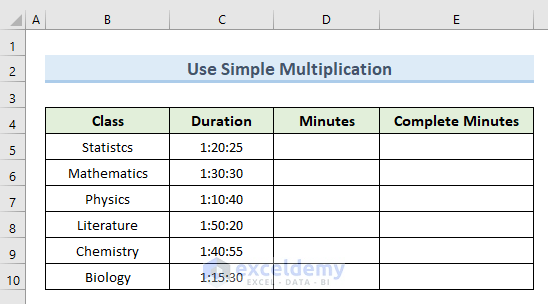So, let’s see the steps regarding this method.

STEPS:

• Firstly, select cell D5. Insert the following formula in that cell:
`=C5*1440`

####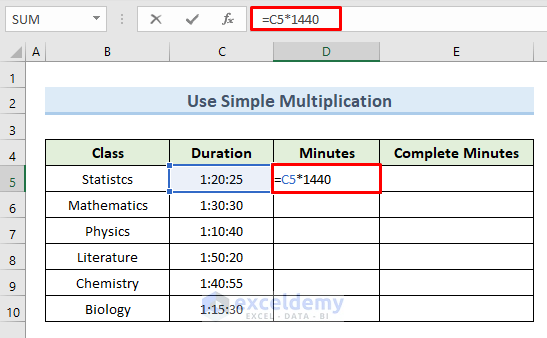• Press Enter. This command returns the decimal value “80.42” minutes.

####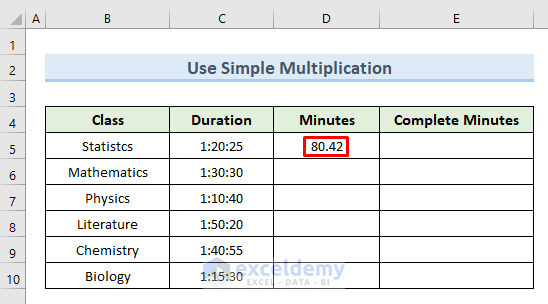• Secondly, move the mouse pointer to the bottom right corner of cell D5 after choosing that cell to make the plus (+) sign visible.

####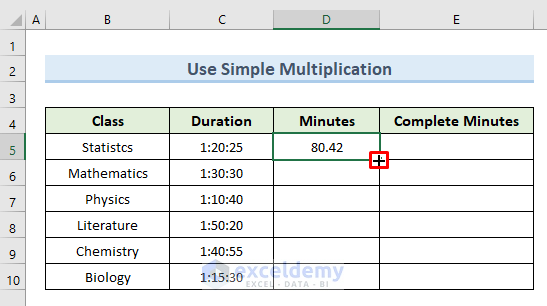• Then drag the Fill Handle down to cell D10 by clicking on the plus (+) sign or double-click on the plus (+).

####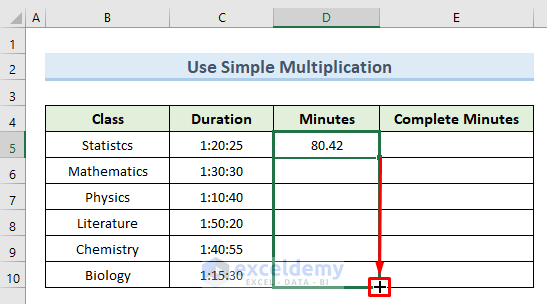• Release the mouse click now.
• As a result, the command above transfers the formula from cell D5 to all other cells. In cells, we can observe the converted decimal values of hours (D5:D10).

####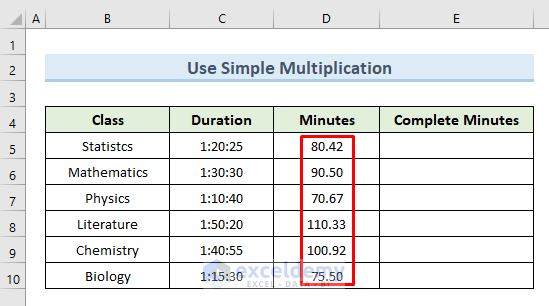• Thirdly, to convert the decimal value into integer value select cell E5 and insert the following formula:
`=INT(D5)`• Press the Enter So, the decimal value of “80.42” minutes has been transformed to an integer value of “80” minutes.

####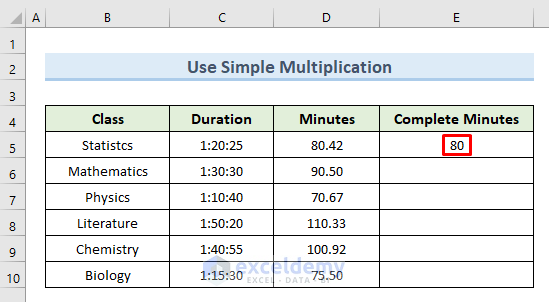• So then, move the mouse cursor to the bottom right corner of cell E5 after selecting that cell to make the plus (+) sign available.

####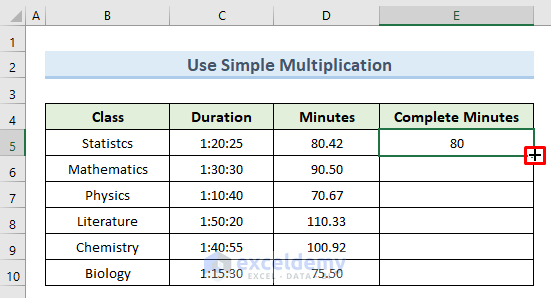• Afterward, drag the Fill Handle down to cell E10 by tapping on the plus (+) sign or do double-click on the plus (+) sign to obtain the same result.

####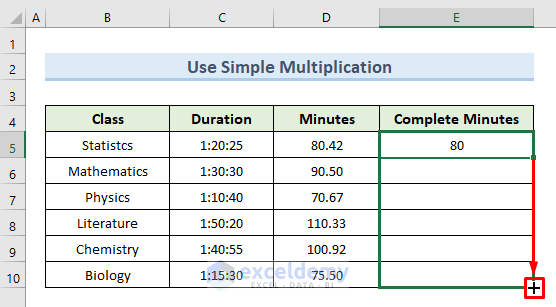• Finally, the integer hours values in cells are determined in cells (E5:E10).

### 2.2 Using Excel CONVERT Function to Convert Minutes to Decimal

The CONVERT function in Microsoft Excel converts a number’s measurement system. We’ll use the CONVERT function in Excel to convert minutes to decimal in this method. We’re going to use the same dataset as in the last example.So, let’s take a look at the steps to perform this action:

STEPS:

• Firstly, select cell D5. Insert the following formula in that cell:
`=CONVERT(C5,"day","mn")`• Hit Enter. This action returns the decimal value “80.42” minutes.• Next, after selecting cell D5, slide the mouse pointer to the bottom right corner of the cell to reveal the plus (+) sign.• Then, clicking the plus (+) sign, move the Fill Handle down to cell D10. We can also double-click on the plus (+) sign to do the same.• Now is the time to free the mouse click.
• As a result, the formula from cell D5 is copied to all other cells using the instruction above. The converted decimal numbers of hours can be seen in cells (D5:D10).• Furthermore, enter the following formula in cell E5 to convert the decimal number to an integer value:
`=INT(D5`)• Press Enter, As a result, the decimal value “80.42” minutes have been converted to an integer “80” minutes.• Again, move the mouse cursor to the bottom right corner of that cell to make the plus (+) sign available after selecting cell E5.• After that, double-click on the plus (+) sign or click on the plus (+) sign to drag the Fill Handle down to cell E10.• At last, the integer hours values are calculated in the cells (E5:E10).### 2.3 Joining Excel HOUR, MINUTE, and SECOND Functions to Convert into Decimal

Multiplying hours by 60 and dividing seconds by the same number is another approach to finding the number of minutes. We will explain this process with the same dataset that we used in the previous example.Let’s see the step-by-step guide to do this method.

STEPS:

• In the beginning, select cell D5 and insert the following formula:
`=HOUR(C5)*60+MINUTE(C5)+SECOND(C5)/60`• Now press Enter. This command returns the minutes value in decimal which is “80.42”.• After that, pick cell D5 and drag the mouse pointer to the cell’s bottom right corner to show the plus (+) sign.• After this drag, the Fill Handle downwards to cell D10 by clicking the plus (+) We may also perform the same thing by double-clicking on the plus (+) sign.• Now free the mouse. click
• As a result, using the instruction above, the formula from cell D5 is duplicated to all other cells. We can see the converted decimal hours in the cells(D5:D10).Here, in the formula, the part “HOUR(C5)*60” converts hours into minutes. The part “SECOND(C5)/60” converts seconds into minutes.

• Additionally, in cell E5, write the following formula to convert the decimal number to an integer value:
`=INT(D5)`• Now, hit Enter. As a consequence, “80.42” minutes have been changed to an integer of “80”.• Now select cell E5 and drop the mouse cursor to the bottom right corner of that cell to display the plus (+).• After that, move the Fill Handle down to cell E10 by clicking on the plus (+) we can do this also by double-clicking on the plus (+) sign.• Finally, the integer minute values are calculated in cells (E5:E10).## Conclusion

In Conclusion, this article explains how to convert hours and minutes to decimal in Excel. Use the practice worksheet that comes with this article to put your skills to the test. If you have any questions, please leave a comment below. Our team will make every effort to respond as quickly as possible. Keep a watch out for more intriguing Microsoft Excel solutions in the future.

## What is ExcelDemy?

ExcelDemy Learn Excel & Excel Solutions Center provides free Excel tutorials, free support , online Excel training and Excel consultancy services for Excel professionals and businesses. Feel free to contact us with your Excel problems.Mukesh Dipto

Hi there! I am Mukesh Dipto. I graduated with a bachelor's degree in engineering. Currently, I am working as a technical content writer in ExcelDemy. You will find all my articles on Microsoft Excel on this site. Outside of the workplace, my hobbies and interests include sports and meeting new people. I also enjoy sports. My favorite sports are football (to watch and play) and Badminton (play).

We will be happy to hear your thoughtsAdvanced Excel Exercises with Solutions PDF